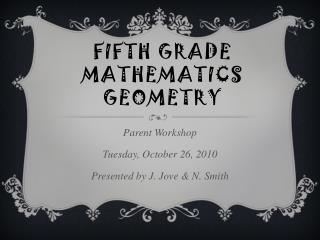DownloadDownload PresentationTélécharger la présentation- - - - - - - - - - - - - - - - - - - - - - - - - - - E N D - - - - - - - - - - - - - - - - - - - - - - - - - - -
##### Presentation Transcript

1. Fifth Grade MathematicsGeometry Parent Workshop Tuesday, October 26, 2010 Presented by J. Jove & N. Smith

2. California Math Standards for Fifth Grade Measurement and Geometry (MG) • Derive & use the formula for the area of a triangle and of a parallelogram by comparing it with the formula for the area of a rectangle (MG 1.1). • Understand the concept of volume and use the appropriate units in measuring systems -- cm³, m, in, yd (MG1.3).

3. Back to the Basics… In order for fifth grade students to be successful in fifth grade math and higher math levels, they must be proficient in multiplying and dividing. This includes multiplying and dividing large numbers. 4 8 6 x 2 1 38,209 ÷ 24

4. Geometry Terms & Symbols • Perpendicular  (lines that intersect each other at right angles)-B & C/B & D • Parallel ‖ (lines that never cross) – C & D • Intersecting Lines (lines that cross; not perpendicular)-A & C • Ray → (a line that goes in one direction from a point) • Line Segment − (the part of a line set off by points) • Line ↔ (a line that goes on in either direction)-A/B/C/D • Congruency  (having the same shape and size) A C D B

5. Measure And Draw Angles Classifying Angles 1 1. Right Angle = 90° 2. Acute Angle = less than 90° 3. Obtuse Angle = larger than 90°, but less than 180° 4. Straight Angle = 180° 2 3 4

6. Triangles(The sum of all angles in a triangle equals 180º) • Here are 4 kinds of triangles: • Isosceles-two congruent sides and angles. • 2) Equilateral-all angles and sides congruent. • 3) Right-one angle equals 90°. • 4) Obtuse-one angle larger than 90°. Equilateral Obtuse Right Isosceles

7. Triangles Continued…(Triangles have more than one name) Acute/ Equilateral Scalene/Obtuse The scalene triangle has no equal sides or angles; the angles in an acute triangle are all less than 90°; the right triangle has one angle equal to 90°; the isosceles triangle has two congruent sides and angles; Right/ Isosceles

8. Quadrilaterals(Four-sided shapes) Angles in any quadrilateral totals to 360. Here are the names of quadrilaterals your child should know: SQUARE (all sides and angles congruent, four right angles) RECTANGLE (opposite sides congruent, four right angles) RHOMBUS (four congruent sides and four congruent angles) TRAPEZOID (top and bottom line are parallel; top angles congruent, bottom angles congruent) PARALLELOGRAM (opposite sides and opposite angles are equal) QUADRILATERAL (four sides, no angles and sides have the same length)

9. Quadrilaterals Continued…(Quadrilaterals also have more than one name) A SQUARE is also a RHOMBUS, a RECTANGLE, aPARRALLELOGRAM, and aQUADRILATERAL ========================== A RECTANGLE is also a PARALLELOGRAM (What else can it be?) ========================= A TRAPEZOID is also a QUADRILATERAL

10. Congruency When shapes are congruent, they have the same shape and same size. Dashes are used to show congruent sides and angles. ( D is  to E)

11. Circles And Angles G A C B E D Area of a Circle: Diameter (AC) Radius (BC or BG) Chord (DE) Center (B) Central Angle (GBC or ABG or B) Point (B)

12. SymmetryRotational & Line Symmetry Rotational Symmetry Line Symmetry

13. Working With Formulas 1) Perimeter = length + length + width + width (the distance around a figure) 2) Area = length x width 3) Area of a Triangle = base x height ÷ 2 4) Area of a Parallelogram = base x height 5) Circumference of a Circle = 3.14 x diameter or 22/7 x diameter ( = 3.14)

14. Volume & Surface Area 4 1 1) Rectangular Prism 2) Cube 3) Cylinder 4) Triangular Prism or Pyramid 2 3

15. Making Connections Did you know that multiplication and division are simply opposites that you can use to check for accuracy? They are related… *In each chapter, students are required to use the four operations: +, −, x, ÷ (Ex. 7x = 49) …In that same way, fractions, decimals, and percentages are all the same; they are just written in different ways.

16. Helpful Websites & ResourcesIf your child continues to struggle, here are some helpful websites: www.eduplace.com www.mrholshoe.com www.themathworksheetsite.com www.ixl.com www.cde.ca.gov www.softschools.com/grades/5thgrade.jsp www.tlsbooks.com/mathworksheets.htm *PARENTS: The State of California website has test released questions that you can use as well!

17. The Importance Of…Homework Believe it or not, teachers do understand that homework is not always easy. But to become better at something, you must practice. We know and believe strongly that parent involvement, support, and a structured environment is essential in helping a student improve and be successful in their classwork, homework, and his/her academic future. That is why we stress that homework be done each night. Students are never given work that they have not been taught. We teach good note-taking skills so that your child can have the tools needed to assist them with their homework. You can help by asking your child to show you his/her notes and homework each day.